# Magic Star Examples

This page contains examples of magic stars from order-5 to order-11. Because there are multiple patterns for each order greater then order-6, there are a total of 16 different magic star examples.
The Magic Star Examples-2 page shows the 14 patterns for orders 12, 13 and 14.

## Contents

 Introduction. . to magic stars Order-5. . . . . # 6 of 12 Order-6. . . . . # 38 of 80 Order-7a. . . . # 47 of 72 Order-7b. . . . # 72 of 72 Order-8a. . . . # 70 of 112 Order-8b. . . . # 45 of 112 Order-9a. . . . # 1615 of 3,014 Order-9b. . . . # 289 of 1,676 Order-9c. . . . # 1676 of 1,676 Order-10a. . . # 10882 of 10,882 Order-10b. . . # 57777 of 115,552 Order-10c. . . # 10882 of 10,882 Order-11a. . . # 27224 of 53 ,528 Order-11b. . . # 41733 of 75,940 Order-11c. . . # 26306 of 53,528 Order-11d. . . # 41733 of 75,940 Magic star Examples-2 ..orders 12, 13, 14

## Example Magic Stars

###Index # 2 of 12 basic solutions. Each of the five lines sum to 24.

This solution has the points also summing to 24.

Because 5 is a prime number, this is a continuous pattern.

Order-5 is the smallest possible magic star. However, it is not a pure magic star because it cannot be formed with the 10 consecutive numbers from 1 to 10. The lowest possible magic sum (24) is formed with the numbers from 1 to 12, leaving out the 7 and the 11.

It is also possible to form 12 basic solutions with the constant 28, by leaving out the 2 and the 6.

###Index # 31  of 80 basic solutions. Each line sums to 26.

This particular pattern has the points also summing to the magic constant of 26. It is one of six solutions that have this property. The six complements of the above, have the valleys summing to 26. It is the only order that can have this property.

Order-6 is the smallest order that can form a pure magic star. It uses the numbers from 1 to 12.

It is the only order (except for order-10A) that has more solutions then a higher order. (Order-7 has only 72 basic solutions.)

Six is the only order that does not have at least one continuous pattern. The pattern consists of two super-imposed triangles.

###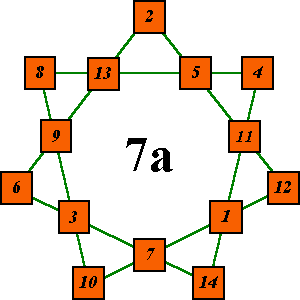Index # 47 of 72 basic solutions. Each line sums to 30.

This particular pattern has all even numbers at the points. Index # 28 is the complement of this one so has the points all odd numbers.

There are 2 star designs for this order, both having the same number of solutions. Order-7 uses the consecutive numbers from 1 to 14
(2 x 7).

Order-7 has less basic solutions then the lower order 6, the only such case (at least to Order-12).

###Index # 72 of 72 basic solutions. Each of the seven lines sum to 30.

This particular solution has the numbers 8 to 14 at the points. Its complement, # 24, has numbers 1 to 7 at the  points.

Each solution contains the consecutive series of integers from 1 to 14. The sum of this series is 105. There are 7 lines with each number appearing in 2 lines, so the magic sum is (2 * 105) / 7 = 30.

Because 7 is a prime number, both designs of this order are continuous, i.e. can be traced without lifting pen from paper.###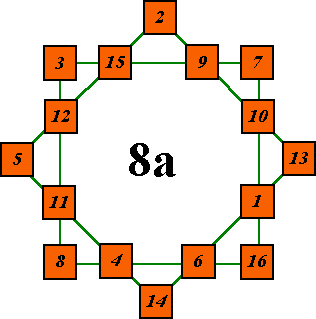Index # 70 of 112 basic solutions. .

Order-8a consists of two super-imposed squares 8 = 2 x 4).
This particular solution has the corners of each square also summing to the constant 34 and is one of eighteen such solutions. In fact, all solutions have the corners of the two squares summing to the same value, which can range from 19 to 49.

Order-8 uses the consecutive  numbers from 1 to 16 (2 x 8). Each of the 8 lines sum to 34

All basic solutions have the lowest point value at the top. The valley to the right of the top point has a lower value then the value to the left of it. Each solution is written as a string of numbers moving along the lines in order, starting from the top point. The solutions are then sorted in ascending order, starting with the first number, to determine the index number.

###Index # 45 of 112 basic solutions. Each line sums to 34.

The points consist of the numbers 1 to 9 with no number 7, for a point total of 38. This is the smallest point total possible. The points of solution number 44 also sum to 38 but it uses the integers 1 to 10, with no 8.

All orders, except order-6 have more then one star pattern. The number of patterns increases by one with each increasing odd order.
Also all orders (except six) have at least one continuous pattern and, if the order is not a prime number, at least one non-continuous pattern.###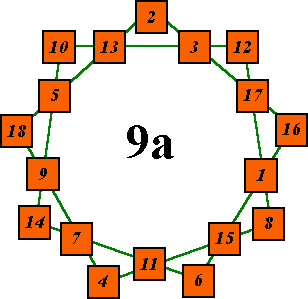Index # 1,615 of 3,014 basic solutions. Each of the 9 lines sum to 38.   It uses the series of numbers from 1 to 18 (2 x 9).

This particular solution has the all even numbers at the points. Its complement, # 995, has all odd numbers at the points. They are 1 of 24 such pairs of solutions for Order-9a. By coincidence, there are also 24 pairs of solutions with all low numbers and all high numbers at the points.

The pattern is continuous. It is one of three star patterns for Order-9, two of which are continuous.###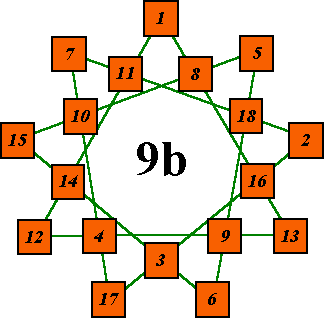This is index # 289 of 1,676 basic solutions.

This pattern is non-continuous. It consists of three super-imposed triangles (9 = 3 x 3). Nine has 1 prime factor pair so has one non-continuous pattern.

This particular solution has the points of each of the three main triangles summing to 26 and is one of 2 such solutions. There are twelve solutions with the three triangles summing to the same value, which ranges from 23 to 34.###Index # 1,676 of 1,676 basic solutions. Each line sums to 38.

Becasue it is at the end of the list, this solution has the nine high number of the series at the points. It's complement, the other member of complement pair # 524, is solution number 671. Naturally, it has the nine low numbers at the points.

Order-9c is a continuous pattern, i.e. it can be traced without lifting pen from paper. All orders have at least one continuous pattern (except order-6), and if the order is a prime number, all the patterns will be continuous.

If the order number is composite, there will be the same number of non-continuous patterns as there are prime factor pairs.###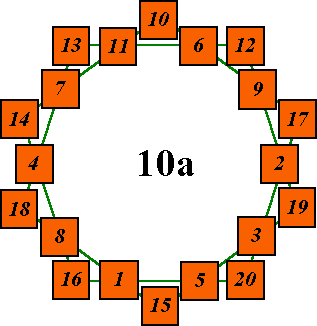Index # 10,882 of 10,882 basic solutions.

It uses the series of numbers from 1 to 20 (2 x 10) with each line summing to 42

This pattern is non-continuous. It consists of two super-imposed pentagons (10 = 2 x 5). In each case, the five points of each pentagon sum to the same value, and the ten cells in each pentagon will sum to the same value because all valleys are common to both pentagons.###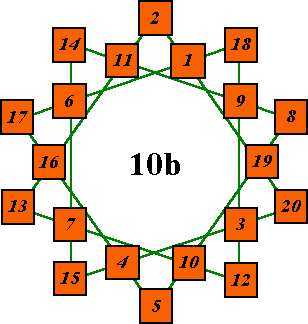Index # 57,777 of 115552 basic solutions.

This pattern is continuous.

Number 57,777  is the first basic solution with a = 2. The last such solution is 84,776,  i.e. exactly 27,000 basic solutions with a = 2.

There is a surprising difference between the number of solutions for Order-10b and the number for patterns a & c. Have I overlooked something? I could find no equivalent solutions and there are exactly 57776 complement pairs (115552/2).

Number of complement pairs = 57,776.
Last solution with a equal to1 = 57,776!

Order-10b has more solutions then order-11 just as order-6 has more then order-7.

###Index # 10,882 of 10,882 basic solutions. Each line sums to 42.

This pattern is non-continuous. It consists of two 5-pointed stars (10 = 2 x 5). In each case, the five points of each star sum to the same value , and the ten cells in each star will sum to the same value because all valleys are common to both stars.###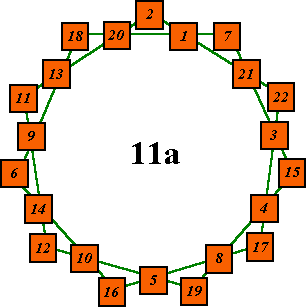Index # 27,224 of 53,528 basic solutions. Each line sums to 46.
Order-11 uses the consecutive numbers from 1 to 22
(2 x 11).

This is the first of four patterns for Order-11. All are continuous because 11 is a prime number.###Index # 41,733 of 75940 basic solutions. Each line sums to 46.

There are 1,670,680 apparently differnet solutions for this pattern.
(11 rotations times 2 reflections times 75,940.)###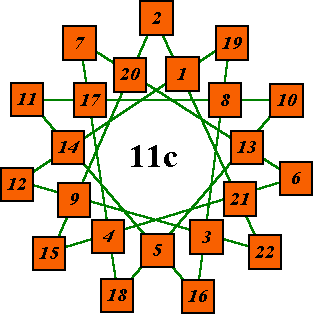Index # 26,306 of 53,528 basic solutions. Each of the 11 lines sums to 46.

This pattern is continuous.

Search times vary widely for different patterns even though all use the same algorithm.
Order-11a = 62 days     Order-11d = 5.3 hours
(using a 200 Mhz Pentium Pro with 32 Megs of memory).###Index # 41,733 of 75,940 basic solutions. Each line sums to 46. This is the first solution starting with the number 2 (position a).

This pattern is continuous because 11 is a prime number.Go to Magic Star Examples-2 to see the 16 order 12, 13 and 14 examples.Harvey Heinz  harveyheinz@shaw.ca